# JP2008129250A - Window changing method for advanced audio coding and band determination method for m/s encoding - Google Patents

## Info

Publication number
JP2008129250A
JP2008129250A JP2006312942A JP2006312942A JP2008129250A JP 2008129250 A JP2008129250 A JP 2008129250A JP 2006312942 A JP2006312942 A JP 2006312942A JP 2006312942 A JP2006312942 A JP 2006312942A JP 2008129250 A JP2008129250 A JP 2008129250A
Authority
JP
Japan
Prior art keywords
window
short
signal
band
long
Prior art date
Legal status (The legal status is an assumption and is not a legal conclusion. Google has not performed a legal analysis and makes no representation as to the accuracy of the status listed.)
Pending
Application number
JP2006312942A
Other languages
Japanese (ja)
Inventor
Yu-Ha Hsiao
Wen-Chieh Lee
Kang-Yen Peng
Keimin Ryu

Original Assignee
National Chiao Tung Univ

Priority date (The priority date is an assumption and is not a legal conclusion. Google has not performed a legal analysis and makes no representation as to the accuracy of the date listed.)
Filing date
Publication date
Application filed by National Chiao Tung Univ, 国立交通大学 filed Critical National Chiao Tung Univ
Priority to JP2006312942A priority Critical patent/JP2008129250A/en
Publication of JP2008129250A publication Critical patent/JP2008129250A/en
Application status is Pending legal-status Critical

• 238000007906 compression Methods 0 abstract 1
• 230000001603 reducing Effects 0 abstract 1

## Images

•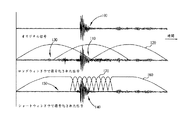•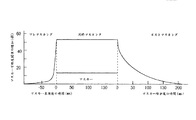••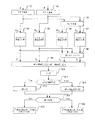•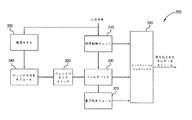•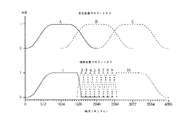•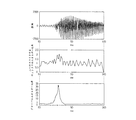•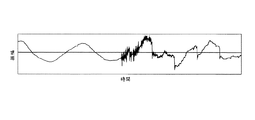•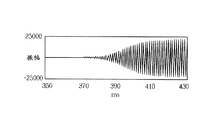•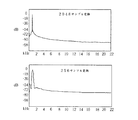•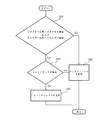••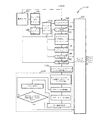•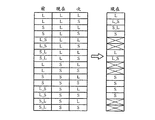•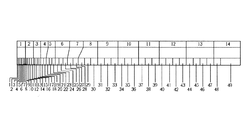•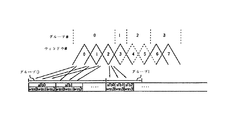•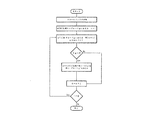•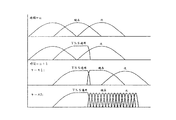•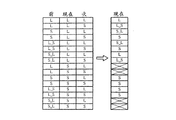•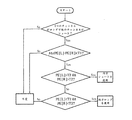•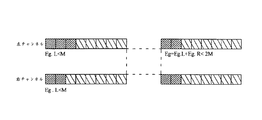•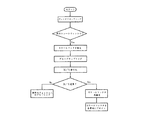•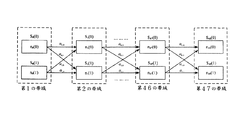•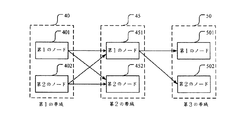•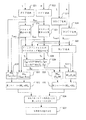## Abstract

An audio compression method for reducing quantization error and a method for determining a band state of M / S coding for AAC are provided.
The present invention provides a method for determining a global energy ratio of a first range of an audio signal and comparing the global energy ratio to a first threshold. The present invention further provides a method for determining a band state of M / S encoding for ACC, the method comprising: receiving at least one audio stream including a majority of the band; a left signal, a right signal, Calculating a first node and a second node of each band including a middle signal and a side signal; calculating a minimum cost path value of each neighboring band; and a state of L / R state or M / Determining the state of each band based on the minimum cost path value that would be in the S state.
[Selection] Figure 25

## Description

The present invention relates to an audio signal, and more particularly, to an improvement in a method for determining a band state of M / S coding for each band for reducing compression error and digital audio coding.

Many digital audio systems rely on signal compression techniques to reduce audio file size. Such audio systems typically sample the raw audio signal using a sample window.
For example, a three minute piece of music is sampled using 1000 sample windows each having a length of 0.18 seconds. The bit resolution of a sample window having a specific length within a normal bit has a significant effect on the quality of the encoded audio signal. For example, if a 0.18 second sample window has 128 bits, each bit corresponds to 0.0013 second of music. These numbers may not match the actual application. Obviously, the higher the number of bits per window, the more quality music is stored, but if there are too many bits, it goes against the purpose of compression. A common digital audio system that uses compression and sample windows is MP3 (Motion Picture Expert Group Audio Layer-3).

The principle of window switching is to change the window size of a filter bank, which is a device for encoding a time-based audio signal into frequency data, and achieves a suitable time-frequency resolution. In general, window switching involves a choice between two predetermined window sizes, large and small. Artificial or unpleasant noise due to compression called pre-echo occurs when a transient signal (eg very short speech) is being encoded. Since transient signals require high coding resolution to accurately represent signal transformations in time, all bit deficiencies allow the quantization error to spread throughout the window period.

To clearly illustrate this problem, FIG. 1 shows an example in which a signal with transient speech is encoded.
In FIG. 1, the original signal 100 to be encoded is shown to have a very small amplitude range that suddenly follows a high amplitude range that follows a small amplitude range. It can be seen that this is a transient signal. After the original signal 100 is encoded by the long window 120, the encoded signal 110 is obtained. Quantization error spread is seen in the encoded signal 110 in the range 130 before the transient high amplitude. Since there is virtually no signal in this range of the original signal 100, the quantization error is not masked by more dominant signals. In general, quantization errors appear and spread when using frequency domain coding over an area where a window contains substantially different amplitudes. As a result of frequency domain compression, the data in the window tends to share features. Quantization errors in the encoded audio are uncomfortable for the listener.

One way to reduce the quantization error is to use windows of different lengths. As shown in FIG. 1, the diffusion of quantization error is reduced in the range 150 of the quantized signal 140 when the long window 160 is used in connection with the short window 170. Compared to the long window encoded signal 110, the diffusion of quantization error is blocked by the short window period of the short window quantized signal.

Furthermore, for current audio coding, M / S (middle signal / side signal) coding is a central technology that effectively reduces inappropriate and redundant information in the stereo channel. For more than two channels, the method used in the current MPEG2 AAC and MPEG4 AAC standards is to divide channels into pairs and then use M / S coding for each pair. When coding gain is present in AAC, the use of M / S coding can be applied to selective spectral domain ranges. In the MPEG4 AAC coding standard, per-band M / S coding provides further flexibility to reduce channel inadequacy and redundancy. However, its flexibility increases encoder design dimensions and complexity.

M / S coding is an expanded auditory audio coding that includes an M / S conversion model that converts L / R (left / right) signals to M / S signals. FIG. 3 is a block diagram showing auditory coding by M / S conversion according to the prior art. The L / R audio signal is divided into overlapping blocks by the analysis filter bank 10 and converted to the frequency domain. If there is a coding gain calculated by the psychoacoustic model 20, the M / S conversion model 15 receives the L / R signal of the converter to the frequency domain and M / S signal. The quantization / encoding model 25 receives signals that quantize and encode these signals along with some parameters determined by the bit allocation 30.

The psychoacoustic model 20 analyzes the L / R signal content and calculates the auditory resolution of the associated human auditory system. Based on the auditory resolution and the available bits, bit allocation 30 determines the preferred quantization method that matches the bit rate. The packing model 35 packs all of the information encoded in the format specified by the standard. There are documents related to M / S coding for each band.

The first document relates to a psychoacoustic model 20 for M / S signals. The psychoacoustic model 20 simulates the human auditory system and tries to give the correct masking threshold for quantization. A masking model of the psychoacoustic model 20 for the L and R channels has already been built in the standard. However, it is not reasonable to put the same procedure on the M and S channels. Moreover, the complexity of the psychoacoustic model 20 contributes to a factor of 15% or more of L / R encoding. The additional complexity from the psychoacoustic model 20 results in an increase in the cost of M / S encoding.

The second document relates to the determination of signal encoding based on each band. This determination relates to the measurement of coding gain from M / S coding to L / R coding. The purpose of switching the band state is to find the maximum coding gain by the psychoacoustic model 20. The best decision is found by evaluating all possible cases, the reconstructed signal is calculated, and the smallest distortion is found from all cases. Since the audio signal firm contains 49 bands, it has a complexity calculation value of instruction O (2 ^ 49) for all possible cases.

M / S coding is freely used, and FAAC, the most representative AAC encoder, has been improved based on Johnston's research with fine parameter adjustments. FIG. 4 is a flowchart showing a process for determining the band state of M / S encoding in FAAC according to the prior art. The psychoacoustic model 20 receives L / R signals that determine the respective band states of M / S encoding and includes the following steps.

Step 1 to Step 2: The left signal and the right signal are converted into a left FFT (L FFT ) signal and a right FFT (R FFT ) signal by Fast Fourier Transform ( FFT ).

Step 3: The left FFT signal and the right FFT signal are converted into a middle FFT (M FFT ) signal and a side FFT (S FFT ) signal.

Step 4 Step 5: Masking Model psychoacoustic model 20 calculates masking threshold value (T L, T R) of the left signal and right signal, respectively.

Step 6 to Step 8: The masking threshold values (T M , T S ) of the middle signal and the side signal are calculated, and the M / S signal is put into a masking model which is the same model in L / R coding, and the masking threshold value is calculated. To get. Thereafter, the final masking threshold is determined by using a binaural MLD (masking level difference) effect.

Step 9 to Step 14: When db <0.25, calculation and comparison are performed to execute Step 15, otherwise, Step 16 is executed.

Step 15: It is determined that the i th band state is the M / S state, and then the M / S conversion model 15 receives the L / R signal of the N th band converter to the M / S signal, and these M / S signals The S signal is quantized and encoded by a quantization / coding model 25.

Step 16: It is determined that the N th band state is the L / R state, and the quantization / coding model 25 receives the N th L / R signal and performs quantization and coding.

There are problems with determining the bandwidth state of FAAC. The first problem is that FAAC uses only the masking threshold dissimilarity that determines M / S band usage, and the M / S signal is put into a masking model that is the same model among the L / R thresholds. To get. Placing the M / S signal is not reasonable. Bandwidth usage can be easily determined by setting the threshold and comparing the criteria, but continuous bandwidth information is not available, and switching of unstable states within one frame is effectively a bit in each bandwidth. Cannot be assigned, and the side information increases. In addition, an optimal bandwidth state determination is found by evaluating all possible cases, calculating the reconstructed signal, and finding the lowest distortion from each case. However, the complexity calculation of the instruction O (2 ^ 49) is too expensive to introduce.

Accordingly, the present invention relates to an audio compression method that reduces quantization errors such as pre-echo, time complexity and other drawbacks, and a method for determining the band state of M / S coding for AAC.
JP-A-8-167878

It is a first object of the present invention to provide a method and related apparatus for reducing quantization error.
The second object of the present invention is to consider each PE (auditory entropy), determine the state of a band for changing the coding state of the adjacent band, and reduce M / for AAC to reduce time complexity. An object of the present invention is to provide a method for determining the band state of S encoding.
A third object of the present invention is to provide a method that finds the optimal bandwidth state determination with simpler and cheaper computation than using any auxiliary function.
The fourth object of the present invention is to provide a method for modifying the M / S coding model of the psychoacoustic model for obtaining the M / S masking threshold, and it is reasonable to put the M / S signal. .
A fifth object of the present invention is to provide a method for determining a band state of M / S coding for AAC, receiving at least one audio stream including a majority band, and a left signal. A first node that is the sum of the PE (auditory entropy) values of the right signal and the left signal, and the sum of the PE values of the middle signal and the side signal in each band including the right signal, the middle signal, and the side signal. calculating a second node, the first node N th band (N + 1) the first or second node of th band, or from the second node of the N th band (N + 1) th first band Or calculating the minimum cost path value of each adjacent band up to the second node and determining the state of each band based on the minimum cost path value that would be in the L / R state or M / S state That comprises the steps, the method provides an inexpensive computing and M / S masking threshold, reduce the time complexity.
Other objects of the invention will become apparent upon reading the description of the best mode for carrying out the invention.

To solve the above problems, the present invention provides a method for determining a global energy ratio of a first range of an audio signal, comparing the global energy ratio with a first threshold, and receiving a block of the audio signal. Determining a global energy ratio of the first range of the audio signal, comparing the global energy ratio with a first threshold, determining a zero cross ratio of the second range of the audio signal, and zero cross ratio Comparing the second and second thresholds, and selecting a short coding window when the global energy ratio or zero crossing ratio exceeds the first or second threshold and no third range tone attack of the audio signal is detected. Steps, the global energy ratio and the zero-cross ratio are the first and Selecting a long encoding window when a threshold of 2 is not exceeded, or when a tone attack of the third range of the audio signal is detected, and the first, second and third in the selected encoding window Encoding a fourth range of the audio signal that is common to the range of.
The present invention further provides a method for determining a band state of M / S coding for ACC, receiving at least one audio stream including a majority of the band, a left signal, a right signal, a middle signal, and Calculating a first node and a second node of each band including a side signal; calculating a minimum cost path value of each adjacent band; and the state is an L / R state or an M / S state Determining a state of each band based on a wax minimum cost path value.

The present invention, from a global energy consideration, allows zero-cross and audio signal tone attacks to select between short and long windows, which can significantly reduce quantization errors.

FIG. 5 is a block diagram illustrating an AAC (advanced audio coding) encoder 300 according to an embodiment of the present invention.
The AAC encoder 300 includes a gain control unit 310, an auditory model 320, a filter bank 330, a window determination module 340, and a bitstream multiplexer 350. Input signals are input from the gain control unit 310 and the auditory model 320 to the AAC encoder 300. The auditory model 320 sends information related to the window determination method (to be described later) to the window determination module 340. The window determination module 340 selects the window size and passes it through the filter bank 330 using the selected window size to encode the appropriate information input signal and code in concert with the output of the gain control unit 310. An audio stream is generated. The AAC encoder 300 further includes a window type switch 360 connected between the window determination module 340 and the filter bank 330 and a quantization module 370 connected between the filter bank 330 and the bitstream multiplexer 350.
The present invention is not limited by the specific embodiments described above, and the AAC encoder 300 may be designed in accordance with the ISO / IEC MPEG-2 / 4 standard.

The filter bank 330 performs a time-frequency transform on the input signal by transitioning between transforms having an input period of 2048 samples or 256 samples by selecting a long window or a short window.

The two window sizes of 2048 samples and 256 samples are merely exemplary, and may be larger than the two window sizes or different size windows. The 256 sample period is for transient signal coding and is a good compromise between frequency selectivity and pre-echo suppression.

As shown in FIG. 1, during the transition between long and short transformations, the bridged transformation between start and stop (ie, start window and stop window) is MDCT (Modified Discrete Cosine Transformation) and IMDCT (inverse). MDCT) is used to maintain time domain aliasing cancellation characteristics and window alignment is maintained. In general, a 2048 sample long transform is called a long sequence, and a 256 sample short transform occurring within a group is called a short sequence. The short sequence is arranged so that about 50% overlaps each other and can have eight short window transformations with half of the boundary transformations overlapping the start and stop windows.
As shown in FIG. 6, these overlapping sequence groups transform windows into start sequences, stop sequences, long sequences and short sequences. The lower curve in FIG. 6 shows the start window following the eight short windows following the stop window, and the upper curve shows the long window encoding in the absence of transient signals.

Since the short window has a high time resolution and the long window has a high frequency resolution, the transient signal benefits from the short window to control the pre-echo effect, and the non-transient signal (ie, no variation) signal is a long window. Analyze signal spectrum lines to get the surplus to benefit from. If a non-transient signal occurs in a short window, the low frequency resolution reduces the accuracy of the frequency domain encoded signal. In the first embodiment, the window determination module 340 of the AAC encoder 300 selects the next window size with reference to the global energy ratio, the zero cross ratio, and the tone attack.

Global energy ratio: Transient signals usually occur when time domain energy changes rapidly. Therefore, energy ratio is used to detect transient signals. Conventional energy ratio detection methods only consider the energy ratio between two sliding short windows, but this energy ratio is unsuitable for detecting signals that increase gradually. In general, the pre-echo effect is generated by the signal portion having the highest energy.

FIG. 7 is a diagram illustrating an example of a speech signal. The three signals in FIG. 7 are, from above, a gradually increasing transient signal, the conventional value of the energy ratio and the global energy ratio according to the present invention. The maximum value of the conventional energy ratio is about 2.1. However, when the transient detection threshold is set to 2.0, erroneous determination easily occurs. The global energy ratio method more easily provides a detectable value of the energy ratio that solves this problem.

In order to determine the energy function En (i) of the 256 sample window Wi, the present invention uses the square sum of the input signal Xk as shown in Equation 1.

(Equation 1)

Then, the highest energy Max_En and the lowest energy Min_En in the set of short window energies En (i) are found. Thus, the global energy ratio is defined as Equation 2.

(Equation 2)

Thus, if the global energy ratio Global_En_Ratio is greater than a predetermined energy threshold, the signal is considered a transient signal. As can be seen from the comparison of the two graphs at the bottom of FIG. 7, Equations 1 and 2 provide improved transient signal detection.

Zero cross ratio: The zero cross rate is used to represent the main frequency content of the signal because the global energy ratio alone cannot detect signals with segments with rapid changes in spectral content.

As an example, FIG. 8 shows a transient signal with a stable global energy ratio, but this signal has an abrupt change in spectral content. When the zero cross rate Ze (i) of each 256 sample short window is defined as Equation 3, the zero cross ratio can detect this type of transient signal.

(Equation 3)

Then, the highest zero cross rate Max_Ze and the lowest zero cross rate Min_Ze within the set of short window zero cross rates are found. Thus, the zero cross ratio is defined as in Equation 4.

(Equation 4)

When the zero cross ratio Ze_Ratio is greater than the zero cross threshold, the signal is considered to be a transient signal. This method is less complex than conventional methods and can accurately detect signal transients in, for example, violins and speech.

Tone attack: In general, a short window has a lower frequency resolution than a long window. FIG. 9 is a diagram illustrating an example of a pure speech signal that is considered to be a transient signal by the global energy ratio of the present invention.
FIG. 10 shows the frequency converted by the 2048 sample conversion (top) and 256 sample conversion (bottom). In FIG. 10, it can be seen that tone signal conversion by the shorter conversion results in an increase in sideband energy. A tone attack effect is defined when the signal has a tone band analyzed by a long window psychoacoustic model (discussed later).

Window determination method: The above-described global energy ratio, zero cross ratio and tone attack are considered in the window determination method. FIG. 11 is a flowchart showing the use of the global energy ratio and the zero-cross ratio for detection of transient signals and avoiding false detection by tone attack analysis. In step 900 it is determined whether either the energy ratio or the zero cross ratio exceeds the respective threshold. If either of these ratios exceeds the threshold, the tone attack is tested at step 910. If both ratios do not exceed the threshold or if a tone attack is detected, a long window is selected at step 920. However, if either of the ratios exceeds the threshold and no tone attack is detected at step 910, a short window is selected at step 930. In the first embodiment, the procedure achieved in the flowchart of FIG. 11 is executed by the window determination module 340 of the AAC encoder 300 shown in FIG.

The above procedure is repeated to complete the encoding of the entire audio signal.

FIG. 12 is a block diagram illustrating an AAC encoder 1000 according to another embodiment of the present invention. Similar to the AAC encoder 300, the AAC encoder 1000 includes an auditory model 320, a filter bank 330, a window determination module 340, and a bitstream multiplexer 350. The AAC encoder 1000 further includes a window type switch 1010, a TNS (temporal noise shaping) unit 1020, a short window scale factor evaluation unit 1030, a grouping unit 1040, and an M / S encoding unit 1050. The AAC encoder 1000 further comprises an iterative loop 1060 that provides gain control.

FIG. 13 is a block diagram illustrating an AAC encoder 1100 according to yet another embodiment of the present invention. Similar to AAC encoder 300, AAC encoder 1100 includes an auditory model 320, a filter bank 330, a window determination module 340, and a bitstream multiplexer 350.

Similar to the AAC encoder 1000, the AAC encoder 1100 further includes a window type switch 1010, a TNS (temporal noise shaping) unit 1020, a short window scale factor evaluation unit 1030, a grouping unit 1040, and an M / S encoding unit 1050. The AAC encoder 1100 further comprises a window coupling unit 1105, a group coupling unit 1110, a short window scale factor reevaluation unit 1120, and an iterative loop 1130 that provides gain control.

Furthermore, although some components representing the procedure are merged, the explanation is divided here for the sake of clarity. For example, the short window scale factor evaluation unit 1030 and the short window scale factor reevaluation unit 1120 can be the same physical device.

Window type switch 360, 1010: After the window determination module 340 determines the window type of the next frame, the current window type uses the window type switch 1010 to compare the next window type with the previous window type. It is switched by.

The start type window is used to bridge a long window and a short window. For this, the window determination module 340 must determine the window type of the next frame in advance, and if the next frame is different from the previous frame, the current frame is switched to the start window type or the stop window type.

FIG. 14 shows an analysis of all possible situations of the window type switch. A long window, a short window, a start window, and a stop window are represented by L, S, L_S, and S_L, respectively. A simple switching equation can be obtained by ignoring some impossible situations.

if (Current == S) {
if (Previous == S || Previous == L_S)
Current = S;
} else {
if (Previous == L || Previous == S_L) {
if (Next == L)
Current = L;
else Current = L_S;
} else if (Previous == S) {
if (Next == L)
Current = S_L;
else
Current = S;
}
}
Previous [] = Current []; Current [] = Next []

This formula is executed by window type switch 360 and / or 1010, and if such a change is required by an adjacent window type, the current window is changed.

Psychoacoustic model: The psychoacoustic model determines which specific speech signals are heard by humans, which are not heard, and controls which speech can be ignored. Different window sizes require different interpretations and standardizations of the psychoacoustic model. If the window sequence is composed of eight short windows, the AAC encoders 300, 1000, 1100 need to execute the short window psychoacoustic model eight times.

The psychoacoustic model calculates the minimum masking threshold required to determine a significant noise level for each band of filter bank 330.

FIG. 15 is a diagram illustrating an example of a mapping result of 49 bands of the long window corresponding to 14 bands of the short window when the sample rate is 44.1 kHz. If the frame uses a short window, SMRs are obtained from the long window.

This refinement is performed by the auditory model 320 or window determination module 340 of the AAC encoders 300, 1000 and 1100.

Grouping unit 1040 and scale factor evaluation unit 1030/1120: If the window sequence consists of eight short windows, the set of 1024 coefficients is actually 8 × representing the time-frequency resolution of the signal over the duration of the eight short windows. It is a matrix of 128 frequency coefficients. Specifically, the set c of 1024 coefficients is indexed as follows before interleaving:

c [g] [w] [b] [k]

g is the group index, w is the index of the window within the group, b is the index of the scale factor band within the window, k is the index of the coefficient within the scale factor band, and the leftmost The index changes most quickly.

After interleaving, the coefficients are indexed as follows:

c [g] [b] [w] [k]

FIG. 16 is a diagram illustrating an example of short window grouping and interleaving. In FIG. 16, group 0 includes short windows indexed as 0, 1 and 2. After interleaving, the first band of these three short windows forms a large scale factor band (sfb 0). The grouping method provides flexibility in the number of scale factor bands for different coding considerations.

The short window can preferably handle the transient signal by controlling the diffusion of quantization noise within the short window. However, when the AAC encoders 1000 and 1100 use short windows, the total number of scale factor bands is twice that when one long window is used.

In the present invention, the grouping method performed by the grouping unit 1040 uses the estimated scale factors of the eight short windows determined by the scale factor estimation unit 1030 or 1120. Accordingly, since the scale factor is estimated by the short window scale factor evaluation unit 1030 which is relatively early in the AAC encoder 1000, the grouping method is more flexible in other codec modules (eg, M / S encoding unit 1050). Applied.

The following equation is used to estimate the scale factor, and the expected ei of the quantization error of the non-uniform quantizer is

(Equation 5)

Delta q is a quantization step size is defined as Equation 6.

(Equation 6)

g is an independent global gain of the scale factor band q. cq is a scale factor of each scale factor band.

The bit factor scale factor estimate is based on a bandwidth proportional noise shaping criterion. The noise level for the scale factor band is proportional to the effective bandwidth B (q).

(Equation 7)

σ 2 N (q) and σ 2 M (q) are noise energy and masking energy associated with the scale factor band q.

In Equation 5, the scale factor is related to the noise power, and Equation 5 and Equation 6 are simply combined. Let E [e i 2 ] = σ 2 N (q) and define T 2 q = σ 2 M (q) · B (q). The prediction of the quantization error for bit allocation is expressed by Equation 8.

(Equation 8)

The square Δ q 2 of the quantization step size is expressed by Equation 9.

(Equation 9)

The difference between the global gain g and the scale factor is evaluated by Equation 10.

(Equation 10)

From Equation 10, the global gain g is evaluated from Equation 11.

(Equation 11)

And scale factors for all sub-bands are obtained.

With respect to grouping methods, the same group of short windows share the scale factor across all scale factor bands in the group, so the shared group's short window shared scale factor (sharesfb g, b ) and estimated scale factor (sf b, w ) differences are limited. In addition to the difference in scale factor, the effect of this difference is proportional to bandwidth ( b ). Therefore, the scale factor error of group g is estimated by Equation 12.

(Equation 12)

The standard of the grouping method minimizes the number of groupings, and the scale factor error Eg of each group becomes smaller than the threshold value M. Based on this criterion, the arithmetic expression shown in the flowchart of FIG. 17 is executed. First, scale factor estimation is performed. Thereafter, the grouping method starts in the first short window. Since a group of short windows is continuous, the arithmetic expression attempts to place each short window in the group to which the previous short window belongs. If the new group's scale factor error is less than the threshold M, the given short window is put into the group. Otherwise, a new group is created for the short window.

TNS unit 1020: TNS is a technique for avoiding the pre-echo phenomenon. This technique is applied in the TNS unit 1020 of the present invention. FIG. 18 is a diagram showing a window type switch configuration when TNS is applied to an attempt to alleviate aliasing. FIG. 19 shows a modified window type switch table for a window type switch 1010 having the following arithmetic expression.

if (Current == S) {
if (Previous == S || Previous == L_S)
Current = S;
} else {
if (Previous == L || Previous == S_L) {
if (Next == L)
Current = L;
else
Current = L_S;
} else if (Previous == S || Previous = L_S) {
if (Next == L)
Current = S_L;
else Current = S;
}
}
Previous [] = Current []; Current [] = Next []

As shown in FIG. 19, when the current window type is long, when the TNS is applied, it is switched to the start window type. At the next time (n + 1), the new situation (when the previous window type is started, the current window type is long, and the next window type is also long) is considered.

M / S encoding unit 1050 and window coupling unit 1105: In stereo encoding, the M / S mechanism is applicable when the window type and grouping method of two stereo channels are the same.

As defined by the MPEG standard, auditory entropy (PE) can assist in determining similarity, as shown in Equation 13.

(Equation 13)

b is the index of the threshold calculation section, E b is the total energy of section b, BW b is the number of frequency lines in section b, and Masking b is the masking of section b.

In order to perform the pre-echo control, the period Masking b is modified as shown in Equation 14.

(Equation 14)

qthr b is the quiet threshold, nb b and nb_l b are the partition thresholds for the current and previous blocks, and repelev is unchanged.

When the signal bursts to high energy, the threshold from nb_l b to nb b increases as a result of the increase in signal energy. Then Masking b is small and PE value is large. When the frame PE becomes higher than a predetermined threshold value PE_SWITCH, the encoder increases the time resolution and changes the window type to short in order to reduce the pre-echo effect.

FIG. 20 is a flowchart showing window coupling. The difference between the left channel PE and the right channel PE is compared with a threshold T1 to determine the similarity. The other PE threshold T2 is used to determine the window type. In general, the above procedure is performed by the M / S encoding unit 1050 and the window coupling unit 1105.

Group coupling unit 1110: For group coupling unit 1110, the sum of the scale factor errors is calculated simultaneously on the channel and the two channels of the group. In the left part of FIG. 21, the grouping method is used individually for the two channels. The purpose of group coupling is to maintain the same grouping configuration in both channels, as shown in the right part of FIG.

The grouping of the present invention minimizes the number of groups and limits the total scale factor error E g for each group of both channels, making it smaller than the new threshold 2M.

FIG. 22 is a flowchart showing window coupling and group coupling, and further shows the relationship with M / S coding. When M / S is turned on, the energy of the two channels is modified and the scale factor associated with each scale factor band is re-estimated. When M / S is not used, the grouping is applied to the two stereo channels separately.

The features of the elements shown in the apparatus of the embodiment of FIGS. 5, 12, and 13 are for clarity of description only.

Furthermore, the present invention also relates to auditory entropy (PE) calculated by the psychoacoustic model, which reflects on the lowest bit required to have a transparent quality evaluated for the left, right and side bands. Is done. The PE value is the simplest way to evaluate bits for the left, right, middle and side signals of the band. The psychoacoustic model then calculates the lowest cost path value for each adjacent band by comparing the PE values from the L / R and M / S bands, and the band state is either the L / R state or the M / S state. To decide.

PE is defined as Equation 15.

(Equation 15)

W i , E i and T i are the bandwidth, energy and masking threshold of the i th band.

To derive the masking threshold for the M / S channel, consider the left and right channels reconstructed as in Equations 16 and 17.

(Equation 16)

(Equation 17)

Equations 18 and 19 are derived from Equations 16 and 17.

(Equation 18)

(Equation 19)

L ′ i [k], R ′ i [k], M ′ i [k] and S ′ i [k] are requantized frequency lines from the decoder. The signal reconstructed due to the quantization error is rewritten as Equations 20 and 21.

(Equation 20)

(Equation 21)

N Li [k], N Ri [k], N Mi [k] and N si [k] are the associated noise for each channel. For transparent audio coding, the difference between N Li [k] and N Ri [k] must be less than the masking threshold for L-band and R-band signals. The difference regarding the partition band is enforced by Equations 22 and 23.

(Equation 22)

(Equation 23)

The sufficient conditions that satisfy the mathematical expressions 22 and 23 that are inequalities are the mathematical expressions 24, 25, and 26.

(Equation 24)

(Equation 25)

(Equation 26)

Therefore, as shown in Equation 27, the threshold is used to replace the threshold directly coming from the M / S signal.

(Equation 27)

For convenience, PEs often use the results communicated from the psychological model FFT. However, the actual encoded signal comes from the result of a modified discrete cosine transform (MDCT) analysis filter bank. Therefore, it is necessary to readjust the masking threshold and change the energy from the FFT format to the MDCT format. The corrected masking threshold is expressed as Equations 28, 29, and 30.

(Equation 28)

(Equation 29)

(Equation 30)

According to Expression 15, PEs in each band in each state are extracted as Expressions 31, 32, 33, and 34.

(Equation 31)

(Expression 32)

(Expression 33)

(Equation 34)

Since all bands PE of L and R, M and S are available, the preferred alternative is chosen after comparing the PEs.

The psychoacoustic model calculates the minimum cost path value of each adjacent band by using the modified Viterbi arithmetic expression, and determines the band state as the L / R state or the M / S state. FIG. 23 is a block diagram showing a modified Viterbi arithmetic expression for minimizing the M / S encoding cost. A trellis is constructed to minimize the cost S k (i) for the end of the k th band where state i and L / R state represent 0 and M / S state represents 1. Each edge represents a transient cost factor for changing the coding state, and each node has its band PE for comparison. The modified Viterbi equation searches for the minimum cost path from the first scale factor band to the end.

Let S k (i) record the minimum accumulated cost of state i from the first band to the k th band, n k (i) represents the i th state node cost of the k th band, and the main Viterbi equation process is This is executed as shown in Equation 35.

(Equation 35)

Q means all state sets, and α i , j represents a transient cost factor. The minimum cost path is found by reversing the tracking path. In other words, the optimal band mode usage can be found by this modified Viterbi arithmetic expression.

To analyze the time complexity, observe that all nodes except the first band node make a comparison only once in each stage.

FIG. 24 is a block diagram showing an embodiment of using the modified Viterbi algorithm of the present invention, comprising a first band 40, a second band 45, and a third band 50, each band being a first band. A node and a second node. The first node 401 of the first band 40 is set to 10, the second node 402 of the first band 40 is set to 20, and the first node 451 of the second band 45 is set to 30. , The second node 452 of the second band 45 is set to 40, the first node 501 of the third band 50 is set to 50, and the second node 502 of the third band 50 is set to 60 Is done.

The transient cost from the first node 401 of the first band 40 to the first node 451 of the second band 45 is set to 1, and the first node 401 of the first band 40 to the second band 45 is set. The transition cost from the second node 452 to the second node 452 is set to 2, the transition cost from the second node 402 in the first band 40 to the first node 451 in the second band 45 is set to 3, The transient cost from the second node 402 of the first band 40 to the second node 452 of the second band 45 is set to 4, and the first node 451 of the second band 45 to the third band 50 The transient cost to the first node 501 is set to 5, and the first node 451 in the second band 45 to the second node 502 in the third band 50 are set to 6. Four cost path values exist between the first band 40 and the second band 45, and two cost path values exist between the second band 45 and the third band 50.

The sum of the first node 401 of the first band 40, the transient cost, and the first node 451 of the second band 45 is the first cost path value, and the first cost path value is 41. The sum of the first node 401 of the first band 40, the transient cost and the second node 452 of the second band 45 is the second cost path value, and the second cost path value is 52. The sum of the second node 402 of the first band 40, the transient cost, and the first node 451 of the second band 45 is the third cost path value, and the third cost path value is 53. The sum of the second node 402 of the first band 40, the transient cost and the second node 452 of the second band 45 is the fourth cost path value, and the fourth cost path value is 64.

The four cost path values are compared to obtain the minimum cost path. The minimum cost path value is 41, and the first node 451 of the second band 45 having the minimum cost path value includes the accumulated value set to 41. Rather than calculating the cost path value from the second node 452 of the second band 45 to the node of the third band 50, the first node 451 of the second band 45 to the node of the third band 50 Calculate the cost path value.

The sum of the accumulated value, the transient cost, and the first node 501 of the third band 50 is the first cost path value, the first cost path value is 96, and the accumulated value is the second cost of the second band 45. Belongs to one node 451. The sum of the accumulated value, the transient cost, and the second node 502 of the third band 50 is the second cost path value, the second cost path value is 107, and the accumulated value is the second band 45. Belongs to one node 451. The two cost path values are compared to obtain a minimum cost path. The minimum cost path value is 96, and the first node 501 of the third band 50 having the minimum cost path value includes a cumulative value. Finally, the minimum cost path is found from the first band 40 to the third band 50.

FIG. 25 is a flowchart showing a method for determining the band state of M / S encoding according to the present invention.

Step 21: The majority of the bands including the left signal are received by the psychoacoustic model, and the left signal is converted into a left FFT signal (L FFT ) by FFT (fast fourier transform).

Step 22: The majority of the bands including the right signal are received by the psychoacoustic model, and the right signal is converted into a right FFT signal (R FFT ) by FFT (fast fourier transform).

Step 23: The left signal is converted into a left MDCT signal (L MDCT ) by MDCT (modified discrete cosine transform) of the analysis filter bank.

Step 24: The right signal is converted into the right MDCT signal (R MDCT ) by MDCT (modified discrete cosine transform) of the analysis filter bank.

Step 25: Calculate middle signal and side signal by using left signal and right signal of the same band.

Step 26: Receive the L FFT signal to calculate the masking threshold (T LFFT ) of the left FFT signal.

Step 27: Receive the R FFT signal to calculate the masking threshold (T RFFT ) of the right FFT signal.

Step 28: Receive the T LFFT signal, T RFFT signal, LFFT signal, RFFT signal, L MDCT signal and R MDCT signal to calculate the masking thresholds (T L , T R ) of the left signal and the right signal, respectively.

Step 29: Receive the TL signal and the TR signal to calculate the masking thresholds (T M , T S ) of the middle signal and the right signal, respectively.

Step 30: Receive the T LFFT signal and the L FFT signal to calculate the PE value (PE L ) of the left signal.

Step 31: Receive the T RFFT signal and the R FFT signal to calculate the PE value (PER) of the right signal.

Step 32: Calculate the first node. The sum of PEL and right PER is the first node.

Step 33: Receive the TM signal and the middle signal to calculate the PE value (PE M ) of the middle signal.

Step 34: Receive the Ts signal and the side signal to calculate the PE value (PEs) of the side signal.

Step 35: Calculate the second node. The sum of the PEM and the right PES is the second node.

Step 36: Calculate the minimum cost path of each adjacent band by the modified Viterbi algorithm.

Step 37: Determine the state of each band based on the minimum cost path value. The state is an L / R state or an M / S state.

When the band state is determined to be the M / S state by the psychoacoustic model, the M / S conversion model receives the L / R signal of the N th band, converts it to the M / S signal, and uses the quantization / coding model The N th band M / S signal is quantized and encoded, otherwise the quantization / coding model receives the N th band L / R signal for quantization and encoding.

The present invention provides a method for determining a band state with an effective calculation method through a band, a PE, and a modified Viterbi equation. The modified Viterbi algorithm can reduce the complexity from O (2 ^ 49) to O (49 * 2) instructions for AAC. Furthermore, the M / S masking threshold is modified to be derived from the L / R psychoacoustic model to obtain the M / S encoding threshold, and it is reasonable to put the M / S signal.

It will be readily apparent that many modifications and variations of these devices and methods may be made during the course of describing the present invention. Accordingly, the above description should be construed as limited only by the following claims.

FIG. 6 shows a signal with encoded transient speech. It is a figure which shows the effect of a different type of masking. It is a block diagram which shows the auditory encoding by M / S conversion in a prior art. It is a flowchart figure which shows the band determination method of M / S encoding of FAAC in a prior art. 1 is a block diagram illustrating an AAC encoder according to the present invention. FIG. FIG. 5 shows a long window encoding and a start-short-stop window sequence. It is a figure which shows the transient signal which increases gradually, the conventional value of an energy ratio, and the global energy ratio by this invention. FIG. 6 shows a transient signal with a stable global energy ratio and abrupt changes in spectral content. It is a figure which shows the example of the signal of a pure audio | voice. FIG. 6 shows the frequency converted by 2048 sample conversion (top) and 256 sample conversion (bottom). It is a flowchart figure which shows the window determination method by this invention. It is a block diagram which shows the 2nd AAC encoder of this invention. It is a block diagram which shows the 3rd AAC encoder of this invention. It is a figure which shows a window type switch table. It is a figure which shows a long-short window psychoacoustic mapping result. It is a figure which shows the example of a short window grouping and interleaving. It is a flowchart figure which shows the short window grouping method of this invention. It is a figure which shows a window type switch structure when TNS is applied. It is a figure which shows the corrected window type switch table when TNS is applied. It is a flowchart figure which shows the window coupling method. It is a figure which shows the example of channel grouping. It is a flowchart figure which shows a window coupling and a group coupling method. It is a block diagram which shows the modified Viterbi arithmetic expression for minimizing M / S encoding cost. FIG. 6 is a block diagram illustrating an example of use of the modified Viterbi algorithm of the present invention. It is a flowchart figure which shows the determination method of the band state of M / S encoding of this invention.

## Claims (37)

1. Receiving a block of audio signals;
Determining a global energy ratio of a first range of the audio signal and comparing the global energy ratio to a first threshold;
Determining a zero cross ratio of a second range of the audio signal and comparing the zero cross ratio to a second threshold;
Selecting a short coding window when either the global energy ratio or the zero crossing ratio exceeds the first or second threshold and no third range tone attack of the audio signal is detected;
Selecting a long encoding window when neither the global energy ratio nor the zero crossing ratio exceeds the first and second thresholds or when a tone attack of the third range of the audio signal is detected;
Encoding a fourth range of the audio signal that is substantially common to the first, second, and third ranges in the selected encoding window. .
2.   2. The audio signal encoding method according to claim 1, wherein the global energy ratio is a ratio of a maximum energy in the first range and a minimum energy in the first range.
3.   The zero cross ratio is a ratio of a zero cross rate of the first sub-range of the second range to a zero cross rate of the second sub-range of the second range, and the zero cross rate of the first sub-range is the second The audio signal encoding method according to claim 1, wherein the zero cross rate of the second sub-range is a minimum value of the second range.
4.   The audio signal encoding method according to claim 1, wherein the tone attack has a tonality higher than a tone threshold.
5.   The global energy ratio is a ratio of the maximum energy of the first range and the minimum energy of the first range, and the zero cross ratio is the zero cross rate of the first sub-range of the second range and the second range. Of the second sub-range, the zero-cross rate of the first sub-range is the maximum value of the second range, and the zero-cross rate of the second sub-range is 2. The audio signal encoding method according to claim 1, wherein the tone attack is a minimum value, and the tone attack has a tonality higher than a tone threshold.
6. The selected window is the next window, the two preselected windows are the current window and the previous window;
Changing the current window to a long to short transition window when the previous window is a long window, the current window is a long window, and the next window is a short window;
Changing the current window from a short to long transition window when the previous window is a short window, the current window is a long window, and the next window is a long window;
Changing the current window to a short window when the previous window is a short window, the current window is a long window, and the next window is a short window;
When the previous window is a short to long transition window, the current window is a long window, and the next window is a short window, changing the current window to a long to short transition window and The audio signal encoding method according to claim 1, further comprising:
7.   2. The audio signal encoding method according to claim 1, further comprising the step of defining a psychoacoustic model of the selected short window as a psychoacoustic model of a corresponding range of the virtual long window.
8. And estimating a scale factor for the short window;
The method of claim 1, further comprising: grouping short windows having a scale factor similar to a predetermined error.
9. And performing M / S encoding on the audio signal;
9. The audio signal encoding method according to claim 8, further comprising the step of re-evaluating the scale factor for the short window.
10. The selected window is the next window, the two preselected windows are the current window and the previous window;
Applying TNS to a fourth range of the audio signal;
Changing the current window to a long to short transition window when the previous window is a long window, the current window is a long window, and the next window is a short window;
Changing the current window from a short to long transition window when the previous window is a short window, the current window is a long window, and the next window is a long window;
Changing the current window to a short window when the previous window is a short window, the current window is a long window, and the next window is a short window;
Changing the current window from a short to long transition window when the previous window is a long to short transition window, the current window is a long window, and the next window is a long window;
Changing the current window to a short window when the previous window is a long to short transition window, the current window is a long window, and the next window is a short window;
When the previous window is a short to long transition window, the current window is a long window, and the next window is a short window, changing the current window to a long to short transition window and The audio signal encoding method according to claim 1, further comprising:
11. The audio signal is a two-channel stereo signal, and
Selecting long or short coding for each channel;
Detecting the difference in the PEs of the two channels when the encoding window size of each channel of the audio signal does not match;
When a difference in PE is detected and the PE for both channels is above the hearing threshold, the short coding window is used for both channels, and when both PEs are below the hearing threshold, the long code is used for both channels. The method according to claim 1, further comprising: using an encoding window.
12.   An AAC encoder comprising a gain control unit, an auditory model, a filter bank, a bitstream multiplexer, and a window determination module programmed to perform the method of claim 1.
13. Receiving a block of audio signals;
Determining a global energy ratio of a first range of the audio signal and comparing the global energy ratio to a first threshold, wherein the global energy ratio is a maximum energy of the first range and a minimum energy of the first range; A step that is a ratio;
Determining a zero cross ratio of a second range of the audio signal and comparing the zero cross ratio with a second threshold, the zero cross ratio being a zero cross rate of a first sub-range of the second range and a second cross-range of the second range; The zero cross rate of the second sub-range, the zero cross rate of the first sub-range is the maximum value of the second range, and the zero cross rate of the second sub-range is the minimum value of the second range. A step and
When either the global energy ratio or the zero crossing ratio exceeds the first or second threshold and no third range tone attack of the audio signal is detected, a short coding window is selected, the tone attack being a tone threshold Selecting a short coding window when having a higher tonality;
Selecting a long encoding window when neither the global energy ratio nor the zero crossing ratio exceeds the first and second thresholds or when a tone attack of the third range of the audio signal is detected;
Encoding a fourth range of the audio signal that is substantially common to the first, second, and third ranges in the selected encoding window. .
14. The selected window is the next window, the two preselected windows are the current window and the previous window;
Changing the current window to a long to short transition window when the previous window is a long window, the current window is a long window, and the next window is a short window;
Changing the current window from a short to long transition window when the previous window is a short window, the current window is a long window, and the next window is a long window;
Changing the current window to a short window when the previous window is a short window, the current window is a long window, and the next window is a short window;
When the previous window is a short to long transition window, the current window is a long window, and the next window is a short window, changing the current window to a long to short transition window and The audio signal encoding method according to claim 13, further comprising:
15.   14. The audio signal encoding method according to claim 13, further comprising the step of defining a psychoacoustic model of the selected short window as a psychoacoustic model of a corresponding range of the virtual long window.
16. And estimating a scale factor for the short window;
The method of claim 13, further comprising: grouping short windows having a scale factor similar to a predetermined error.
17. And performing M / S encoding on the audio signal;
17. The audio signal encoding method according to claim 16, further comprising the step of re-evaluating the scale factor for the short window.
18. The selected window is the next window, the two preselected windows are the current window and the previous window;
Applying TNS to a fourth range of the audio signal;
Changing the current window to a long-to-short transition window when the previous window is a long window, the current window is a long window, and the next window is a short window;
Changing the current window from a short to long transition window when the previous window is a short window, the current window is a long window, and the next window is a long window;
Changing the current window to a short window when the previous window is a short window, the current window is a long window, and the next window is a short window;
Changing the current window from a short to long transition window when the previous window is a long to short transition window, the current window is a long window, and the next window is a long window;
Changing the current window to a short window when the previous window is a long to short transition window, the current window is a long window, and the next window is a short window;
When the previous window is a short to long transition window, the current window is a long window, and the next window is a short window, the step of changing the current window to a long to short transition window and 14. The audio signal encoding method according to claim 13, further comprising:
19. The audio signal is a two-channel stereo signal, and
Selecting long or short coding for each channel;
Detecting the difference in the PEs of the two channels when the encoding window size of each channel of the audio signal does not match;
When a difference in PE is detected and the PE for both channels is above the hearing threshold, the short coding window is used for both channels, and when both PEs are below the hearing threshold, the long code is used for both channels. The method of claim 13, further comprising the step of using an encoding window.
20.   An AAC encoder comprising a gain control unit, an auditory model, a filter bank, a bitstream multiplexer and a window determination module programmed to perform the method of claim 13.
21. Receiving at least one audio stream having a majority of bands, each band having a left signal and a right signal;
Calculating a middle signal and a side signal by using a left signal and a right signal in the same band; and
Calculating a first node that is the sum of the PE values of the left signal and the right signal and a second node that is the sum of the PE values of the middle signal and the side signal for each band;
Each is from a first node N th band until (N + 1) the first or second node of th band, or from the second node of the N th band (N + 1) th first or second node of the band Calculating the minimum cost path value of the adjacent band;
Determining a state of each band based on a minimum cost path value where the state may be an L / R state or an M / S state, and a band state of M / S encoding for AAC, comprising: Decision method.
22. And calculating a minimum cost path value, said step comprising:
Calculating a majority of cost path values where each cost path value is from a first band node to a second band node;
22. The method for determining a band state of M / S encoding for AAC according to claim 21, further comprising: obtaining a minimum cost path value by comparing cost path values.
23.   The audio stream includes four cost path values between a first band and a second band and two cost path values between the remaining adjacent bands of the audio stream. Of determining the band state of M / S coding for AAC of the first.
24. And calculating a minimum cost path value between the first band and the second band, said step comprising:
Calculating each cost path value by using the sum of the first band node, the transient cost and the second band node;
24. The method for determining a band state of M / S encoding for AAC according to claim 23, further comprising: obtaining a minimum cost path value by comparing cost path values.
25. And calculating a minimum cost path value between the N th band of the remaining adjacent bands and the (N + 1) th band, said step comprising:
Calculating each cost path value by using the cumulative value, the transient cost and the sum of the nodes in the (N + 1) th band;
24. The method for determining a band state of M / S encoding for AAC according to claim 23, further comprising: obtaining a minimum cost path value by comparing cost path values.
26. The accumulated value, (N-1) th band and M / S code for AAC according to claim 25, characterized in that belonging to the node of the N th band with a least-cost path between the N th band How to determine the bandwidth state of a network.
27. Further, the method includes calculating a minimum cost path value, the step comprising:
The method for determining a band state of M / S coding for AAC according to claim 21, further comprising: calculating a minimum cost path value of each adjacent band of the audio stream by a modified Viterbi arithmetic expression. .
28. And calculating a minimum cost path value, said step comprising:
Calculating a majority of cost path values where each cost path value is from a first band node to a second band node;
The method for determining the band state of M / S encoding for AAC according to claim 27, comprising: comparing a cost path value to obtain a minimum cost path value.
29.   28. The audio stream includes four cost path values between a first band and a second band and two cost path values between the remaining adjacent bands of the audio stream. Of determining the band state of M / S coding for AAC of the first.
30. And calculating a minimum cost path value between the first band and the second band, said step comprising:
Calculating each cost path value by using the sum of the first band node, the transient cost and the second band node;
30. The method for determining a band state of M / S encoding for AAC according to claim 29, comprising: comparing a cost path value to obtain a minimum cost path value.
31. And calculating a minimum cost path value between the N th band of the remaining adjacent bands and the (N + 1) th band, said step comprising:
Calculating each cost path value by using the cumulative value, the transient cost and the sum of the nodes in the (N + 1) th band;
30. The method for determining a band state of M / S encoding for AAC according to claim 29, comprising: comparing a cost path value to obtain a minimum cost path value.
32. The accumulated value, (N-1) th band and M / S code for AAC according to claim 31, characterized in that belonging to the node of the N th band with a least-cost path between the N th band How to determine the bandwidth state of a network.
33. And calculating the PE value of the left signal and the right signal, said step comprising:
Converting left and right signals into left and right FFT signals by FFT;
Receiving a left FFT signal and a right FFT signal to calculate a masking threshold for the left FFT signal and the right FFT signal;
22. The M / S encoding for AAC according to claim 21, comprising receiving a masking threshold, a left FFT signal and a right FFT signal to calculate PE values of the left signal and the right signal, respectively. How to determine the bandwidth status of
34. In addition, before calculating the middle and side signals,
The method of claim 21, further comprising: converting left and right signals into left and right MDCT signals by MDCT and calculating middle and side signals. Bandwidth determination method.
35. The method further includes the step of calculating the PE value of the middle signal and the side signal,
Calculating a middle signal and a side signal masking threshold;
35. The method of M / S encoding for AAC according to claim 34, further comprising: receiving a masking threshold, a middle signal and a side signal to calculate a PE value of the middle signal and the side signal, respectively. Bandwidth determination method.
36. Calculating a middle signal and side signal masking thresholds, said steps comprising:
Converting left and right signals into left and right MDCT signals by MDCT; converting left and right signals into left and right FFT signals by FFT; and
Receiving left and right FFT signals to calculate left and right FFT signal masking thresholds; and calculating left and right FFT signal masking thresholds to calculate left and right FFT masking thresholds. Receiving a masking threshold, a left FFT signal, a right FFT signal, a left MDCT signal and a right MDCT signal;
36. The M / S coding for AAC according to claim 35, comprising: receiving a masking threshold for the left signal and the right signal to calculate a masking threshold for the middle signal and the right signal, respectively. Bandwidth determination method.
37.   The band state of M / S encoding for AAC according to claim 36, wherein the masking threshold values of the middle signal and the side signal are respectively set to half the minimum value of the masking threshold values of the left signal and the right signal. How to determine.
JP2006312942A 2006-11-20 2006-11-20 Window changing method for advanced audio coding and band determination method for m/s encoding Pending JP2008129250A (en)

## Priority Applications (1)

Application Number Priority Date Filing Date Title
JP2006312942A JP2008129250A (en) 2006-11-20 2006-11-20 Window changing method for advanced audio coding and band determination method for m/s encoding

## Applications Claiming Priority (1)

Application Number Priority Date Filing Date Title
JP2006312942A JP2008129250A (en) 2006-11-20 2006-11-20 Window changing method for advanced audio coding and band determination method for m/s encoding

## Publications (1)

Publication Number Publication Date
JP2008129250A true JP2008129250A (en) 2008-06-05

# Family

## Family Applications (1)

Application Number Title Priority Date Filing Date
JP2006312942A Pending JP2008129250A (en) 2006-11-20 2006-11-20 Window changing method for advanced audio coding and band determination method for m/s encoding

## Country Status (1)

JP (1) JP2008129250A (en)

## Cited By (2)

* Cited by examiner, † Cited by third party
Publication number Priority date Publication date Assignee Title
CN104538041A (en) * 2014-12-11 2015-04-22 深圳市智美达科技有限公司 Method and system for detecting abnormal sounds
JP2018513402A (en) * 2015-03-09 2018-05-24 フラウンホッファー−ゲゼルシャフト ツァ フェルダールング デァ アンゲヴァンテン フォアシュンク エー．ファオ Apparatus and method for encoding or decoding multi-channel signals

## Citations (3)

* Cited by examiner, † Cited by third party
Publication number Priority date Publication date Assignee Title
JPH02259699A (en) * 1989-03-30 1990-10-22 Sharp Corp Sound recording and reproducing device
JPH08179794A (en) * 1994-12-21 1996-07-12 Sony Corp Sub-band coding method and device
JP2000004163A (en) * 1998-06-16 2000-01-07 Matsushita Electric Ind Co Ltd Method and device for allocating dynamic bit for audio coding

## Patent Citations (3)

* Cited by examiner, † Cited by third party
Publication number Priority date Publication date Assignee Title
JPH02259699A (en) * 1989-03-30 1990-10-22 Sharp Corp Sound recording and reproducing device
JPH08179794A (en) * 1994-12-21 1996-07-12 Sony Corp Sub-band coding method and device
JP2000004163A (en) * 1998-06-16 2000-01-07 Matsushita Electric Ind Co Ltd Method and device for allocating dynamic bit for audio coding

## Cited By (3)

* Cited by examiner, † Cited by third party
Publication number Priority date Publication date Assignee Title
CN104538041A (en) * 2014-12-11 2015-04-22 深圳市智美达科技有限公司 Method and system for detecting abnormal sounds
JP2018513402A (en) * 2015-03-09 2018-05-24 フラウンホッファー−ゲゼルシャフト ツァ フェルダールング デァ アンゲヴァンテン フォアシュンク エー．ファオ Apparatus and method for encoding or decoding multi-channel signals
US10388289B2 (en) 2015-03-09 2019-08-20 Fraunhofer-Gesellschaft Zur Foerderung Der Angewandten Forschung E.V. Apparatus and method for encoding or decoding a multi-channel signal

## Similar Documents

Publication Publication Date Title
ES2526767T3 (en) Audio encoder, procedure to encode an audio signal and computer program
CN101371447B (en) Complex-transform channel coding with extended-band frequency coding
TWI307248B (en) Apparatus and method for generating multi-channel synthesizer control signal and apparatus and method for multi-channel synthesizing
AU2006270259B2 (en) Selectively using multiple entropy models in adaptive coding and decoding
ES2677900T3 (en) Encoder and audio decoder
US7240001B2 (en) Quality improvement techniques in an audio encoder
US7668711B2 (en) Coding equipment
RU2387024C2 (en) Coder, decoder, coding method and decoding method
KR101120913B1 (en) Apparatus and method for encoding a multi channel audio signal
US6766293B1 (en) Method for signalling a noise substitution during audio signal coding
US7548855B2 (en) Techniques for measurement of perceptual audio quality
US20060093048A9 (en) Partial Spectral Loss Concealment In Transform Codecs
US20040196913A1 (en) Computationally efficient audio coder
KR100346066B1 (en) Method for coding an audio signal
KR101209410B1 (en) Analysis filterbank, synthesis filterbank, encoder, decoder, mixer and conferencing system
US20070016427A1 (en) Coding and decoding scale factor information
ES2307188T3 (en) Multichannel synthesizer and procedure to generate a multichannel output signal.
JP3263168B2 (en) Method and decoder for encoding an audible sound signal
EP1904999B1 (en) Frequency segmentation to obtain bands for efficient coding of digital media
US9305558B2 (en) Multi-channel audio encoding/decoding with parametric compression/decompression and weight factors
JP4425148B2 (en) Reduction of scale factor transmission costs for MPEG-2 Advanced Audio Coding (AAC) using lattice-based post-processing techniques
TWI397903B (en) Economical loudness measurement of coded audio
US7761290B2 (en) Flexible frequency and time partitioning in perceptual transform coding of audio
US20070016404A1 (en) Method and apparatus to extract important spectral component from audio signal and low bit-rate audio signal coding and/or decoding method and apparatus using the same
US7460993B2 (en) Adaptive window-size selection in transform coding

## Legal Events

Date Code Title Description
RD02 Notification of acceptance of power of attorney

Free format text: JAPANESE INTERMEDIATE CODE: A7422

Effective date: 20090828

A131 Notification of reasons for refusal

Free format text: JAPANESE INTERMEDIATE CODE: A131

Effective date: 20100315

A02 Decision of refusal

Free format text: JAPANESE INTERMEDIATE CODE: A02

Effective date: 20101201